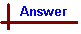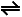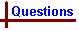Solubility Equilibrium Link to Data Page Your feedback on these self-help problems is appreciated. Click here to send an e-mail. Shortcut to Questions Q: 1 2 3 4 5 6 7 8 9 10 11 1 Silver acetate is a sparingly soluble salt. How is its solubility affected by the addition of the following substances to a saturated solution containing some solid? Write "increased", "decreased", or "no change". Give a justification for your answer in each case. (1.) Sodium acetate (2.) Sodium chloride (3.) Nitric acid (4.) Potassium cyanide2 At 298 K the water solubility of magnesium hydroxide is 1.26 x 10-2 gram litre-1. Calculate the solubility product constant of magnesium hydroxide at this temperature.3 At a certain temperature the solubility of lead(II) sulfate in water is 1.25 x 10-4 M. Calculate the solubility product constant of lead(II) sulfate at this temperature.4 The concentration of Ag+ ion in a saturated solution of silver oxalate (Ag2C2O4) at a certain temperature is 2.2 x 10-4 M. Calculate the solubility product constant of silver oxalate at this temperature.5 Using Ksp values, calculate the molar solubility of both salts in each of the following pairs of insoluble salts. Also state which is the more soluble salt of each pair. (1.) Fe(OH)2, Fe(OH)3 (2.) Ag2SO4, SrCrO46 Find the solubility at 298 K of PbSO4 in: (1.) pure water (2.) 0.10 M lead(II) nitrate (3.) 0.10 M sodium sulfate7 At 298 K the solubility of barium sulfate in water is 1 x 10-5 M. What is its solubility in 0.1 M potassium sulfate at 298 K?8 For each of the following, decide whether or not a precipitate could be expected to form at 298 K: (1.) Silver nitrate (0.0050 g) is added to 0.0010 M NaCl (2.0 litre) (2.) Calcium nitrate (2.0 x 10-3 g) and sodium carbonate (6.0 x 10-3 g) are dissolved in water (500 ml). (3.) 0.0010 M magnesium nitrate (50 ml) is added to 0.0010 M sodium hydroxide (200 ml).9 A metal from Periodic Group 2 is converted to the hydroxide by reaction with water. The saturated solution of this hydroxide has a pH of 9.60. What is the concentration of metal ions in this solution?10 The [Ag+] of a solution is 4.0 x 10-3 M. Calculate the [Cl-] that must be exceeded before silver chloride can precipitate at 298 K.11 (1.) A concentrated water solution of sodium carbonate is added slowly with stirring to a solution that contains 0.10 M calcium ion and 0.10 M magnesium ion. What is the first solid to precipitate? (2.) Neglecting dilution, what is the concentration of carbonate ion in the reaction mixture at the instant when a precipitate first appears? (3.) Neglecting dilution, what is the concentration of calcium ion in solution at the instant when a precipitate of magnesium carbonate first appears?Solubility Equilibrium (Answers) 1 The dissolution of silver acetate in water can be described as an equilibrium: CH3COOAg(s)CH3COO-(aq) + Ag+(aq) Like other equilibria it is subject to LeChatelier's principle, which qualitatively describes the effect of concentration changes in the system. There are two possible ways an added species can effect solubility. If one of the species present in the dissolution equation is added it will have a direct effect on the equilibrium, eg. part (1.). Alternatively, if an added species can react with one of the species present in the dissolution equilibrium there may be an indirect effect on the dissolution equilibrium (eg. parts 2, 3 and 4). In either case, the direction the dissolution equlibrium is forced will determine if solubility is increased or decreased. (1.) The addition of sodium acetate will result in a decrease in solubility. It is evident from the dissolution equation that increasing [CH3COO-] will shift the equilibrium to the left, hence favouring solid CH3COOAg (ie an effective decrease in solubility). This is known as the common ion effect. (2.) AgCl is less soluble than CH3COOAg, so Cl- withdraws Ag+ from solution more than CH3COO- Ag+(aq) + Cl-(aq)AgCl(s) Referring to the dissolution equation, removing Ag+ ions will shift the equilibrium to the right, hence increasing the solubility of CH3COOAg. (3.) Nitric acid reacts with the acetate ion to produce acetic acid: H+ + CH3COO- → CH3COOH Acetic acid is a weak acid, so does not dissociate to H+ and CH3COO- to a great extent. As a result, the addition of nitric acid effectively reduces [CH3COO-], hence shifting the equilibrium to the right, increasing the solubility of CH3COOAg. (4.) The cyanide ion reacts with Ag+ to form the dicyanoargentate(I) ion: Ag+ + 2CN-[Ag(CN)2]- This reaction lowers [Ag+], and hence forces the equilibrium to the right, increasing the solubility of CH3COOAg.2 Mg(OH)2 dissolves in water to a small extent according to the following equation: Mg(OH)2(s)Mg2+ + 2OH- KSP Mg(OH)2 = [Mg2+] [OH-]2 Note that in the expression for KSP, [OH-] is raised to the power of 2 since two OH- ions are produced as the equation is written. No. mol Mg(OH)2 in 1.26 x 10-2 g = 1.26 x 10-2 g / 58.33 g mol-1 = 2.16 x 10-4 mol. [Mg2+] = 2.16 x 10-4 mol L-1 [OH-] = 4.32 x 10-4 mol L-1 ∴ KSP = (2.16 x 10-4 mol L-1) x (4.32 x 10-4 mol L-1)2 = 4.03 x 10-11 mol3 L-33 PbSO4 dissolves to a small extent in water according to the following equation: PbSO4(s)Pb2+ + SO42- KSP PbSO4 = [Pb2+] [SO42-] No. mol PbSO4 in 1.25 x 10-4 mol [Pb2+] = 1.25 x 10-4 mol L-1 [SO42-] = 1.25 x 10-4 mol L-1 ∴ KSP = (1.25 x 10-4 mol L-1) x (1.25 x 10-4 mol L-1) = 1.56 x 10-8 mol2 L-24 Ag2C2O4 dissolves in water to a small extent according to the following equation: Ag2C2O4(s)2Ag+ + C2O42- KSP = [Ag+]2 [C2O42-] From the above equation it is evident that [C2O42-] = 0.5 x [Ag+]. Substituting into the equation for KSP: KSP = [Ag]2 x 0.5 x [Ag+] = (2.2 x 10-4 mol L-1)2 x 0.5 x (2.2 x 10-4 mol L-1) = 5.3 x 10-12 mol3 L-35 (1.) Fe(OH)2(s)Fe2+ + 2OH- From the data sheet, KSP Fe(OH)2 = 8 x 10-16 = [Fe2+] [OH-]2 Let the molar solubility of Fe(OH)2 be x. Now [Fe2+] = x, [OH-] = 2x. These figures are arrived at by considering that one mole Fe(OH)2 dissolves to give one mole Fe2+ and two moles OH-. ∴ KSP = (x)(2x)2 = 4x3 = 8 x 10-16 mol3 L-3 ∴ Molar solubility of Fe(OH)2 = x = 6 x 10-6 mol L-1 Fe(OH)3(s)Fe3+ + 3OH- From the data sheet, KSP Fe(OH)3 = 4 x 10-40 = [Fe3+] [OH-]3 Let the molar solubility of Fe(OH)3 be x. Now [Fe2+] = x, [OH-] = 3x ∴ KSP = (x)(3x)3 = 27x4 = 4 x 10-40 mol4 L-4 ∴ Molar solubility of Fe(OH)2 = x = 6 x 10-11 mol L-1 Therefore Fe(OH)2 is the more soluble salt. (2.) Ag2SO4(s)2Ag+ + SO42- From the data sheet: KSP Ag2SO4 = 2 x 10-5 = [Ag+]2 [SO42-] Let the molar solubility of Ag2SO4 be x. Now [Ag+] = 2x, [SO42-] = x ∴ KSP = (2x)2(x) = 4x3 = 2 x 10-5 mol3 L-3 ∴ Molar solubility of Ag2SO4 = x = 2 x 10-2 mol L-1 SrCrO4(s)Sr2+ + CrO42- From the data sheet: KSP SrCrO4 = 2 x 10-5 = [Sr2+] [CrO42-] Let the molar solubility of SrCrO4 be x. Now [Sr2+] = x, [CrO42-] = x ∴ KSP = x2 = 2 x 10-5 mol2 L-2 ∴ Molar solubility of Ag2SO4 = x = 4 x 10-3 mol L-1 Ag2SO4 is the more soluble.6 (1.) PbSO4(s)Pb2+ + SO42- From the data sheet, KSP PbSO4 = 2 x 10-8 = [Pb2+] [SO42-] Let the molar solubility of PbSO4 be x Now [Pb2+] = x, [SO42-] = x ∴ KSP = x2 = 2 x 10-8 mol2 L-2 ∴ Molar solubility of PbSO4 = x = 1 x 10-4 mol L-1 (2.) The addition of Pb(NO3)2 increases [Pb2+], and effects the equilibrium (the common ion effect). KSP PbSO4 = 2 x 10-8 = [Pb2+] [SO42-] Upon addition of Pb(NO3)2, [Pb2+] equals 0.10 mol L-1 (this is ignoring the relatively tiny contribution from the dissolved lead sulfate). Again, we let the molar solubility of PbSO4 = x Now [Pb2+] = 0.10 mol L-1, [SO42-] = x KSP = (0.10 mol L-1) x (x) = 2 x 10-8 mol2 L-2 ∴ Molar solubility of PbSO4 = x = 2 x 10-7 mol L-1 Note that this figure is 1 / 500 the value for pure water, due to the common ion effect. (3.) The addition of Na2SO4 increases [SO42-] and affects the equilibrium. KSP PbSO4 = 2 x 10-8 = [Pb2+] [SO42-]. Upon addition of Na2SO4 , [SO42-] equals 0.10 mol L-1 (this is ignoring the relatively tiny contribution from the dissolved lead sulfate). Again, we let the molar solubility of PbSO4 = x. Now [Pb2+] = x, [SO42-] = 0.10 mol L-1 KSP = (x) x (0.10 mol L-1) = 2 x 10-8 mol2 L-2 ∴ Molar solubility of PbSO4 = x = 2 x 10-7 mol L-17 KSP BaSO4 = 1 x 10-10 mol2 L-2 = [Ba2+] [SO42-] Let the molar solubility of BaSO4 be x. [Ba2+] = x [SO42-] = 0.10 M KSP = (x) x (0.10 M) ∴ Molar solubility of BaSO4 = x = 1 x 10-8 mol L-18 (1.) AgNO3 + NaClAgCl + NaNO3 Total volume of solution = 2.0 L [Cl-] = 0.0010 mol L-1 No. mol AgNO3 = 0.0050 g / 169.91 g mol-1 = 2.94 x 10-5 mol ∴ [Ag+] = 1.47 x 10-5 mol L-1 KSP AgCl = [Ag+] [Cl-] = 2 x 10-10 mol2 L-2 from data sheet. In the solution, [Ag+] [Cl-] = (1.47 x 10-5 mol L-1) (0.0010 mol L-1) = 1.47 x 10-8 mol2 L-2. This value is greater than the data sheet value of KSP, so a precipitate will form. (2.) Ca(NO3)2 + Na2CO3CaCO3 + 2NaNO3 Total volume of solution = 500 mL No. mol Ca(NO3)2 = 2.0 x 10-3 g / 164.12 g mol-1 = 1.22 x 10-5 mol ∴ [Ca2+] = 2.44 x 10-5 mol L-1 No. mol Na2CO3 = 6.0 x 10-3 g / 105.99 g mol-1 = 5.66 x 10-5 mol ∴ [CO32-] = 1.13 x 10-4 mol L-1 KSP CaCO3 = [Ca2+] [CO32-] = 5 x 10-9 mol2 L-2 from data sheet. In the solution, [Ca2+] [CO32-] = (2.44 x 10-5 mol L-1) (1.13 x 10-4 mol L-1) = 2.76 x 10-9 mol2 L-2. This value is less than the data sheet value of KSP, so a precipitate will not form. (3.) Mg(NO3)2 + 2NaOHMg(OH)2 + 2NaNO3 Total volume of solution = 250 mL, so the concentration of each species becomes: [Mg2+] = 2 x 10-4 mol L-1 [OH-] = 8 x 10-4 mol L-1 KSP Mg(OH)2 = [Mg2+] [OH-]2 = 1 x 10-11 mol3 L-3 from data sheet. In the solution, [Mg2+] [OH-]2 = (2 x 10-4 mol L-1) (8 x 10-4 mol L-1)2 = 1.28 x 10-10 mol2 L-2. This value is more than the data sheet value of KSP, so a precipitate will form.9 Since the metal is from group 2, it must have a valency of 2. Therefore the hydroxide will have the formula M(OH)2, using M to denote the symbol for the element. pH = 9.60 ∴ pOH = 14.00 - 9.60 = 4.40 [OH-] = 10-4.40 = 3.98 x 10-5 mol L-1 From the empirical formula we know there is one M2+ ion for every two OH- ions, ∴ [M2+] = [OH-] / 2 = 2.0 x 10-5 mol L-110 KSP(AgCl) = 2 x 10-10 = [Ag+] [Cl-] [Ag+] = 4.0 x 10-3 mol L-1 Minimum [Cl-] required for precipitate to form = KSP / [Ag+] = 2 x 10-10 mol2 L-2 / 4.0 x 10-3 mol L-1 = 5 x 10-8 mol L-1 Therefore, when [Cl-] exceeds 5 x 10-8 mol L-1 AgCl will precipitate.11 (1.) KSP(CaCO3) = 5 x 10-9 mol2 L-2 = [Ca2+] [CO32-] [Ca2+] = 0.10 mol L-1 Minimum [CO32-] required for precipitate to form = KSP / [Ca2+] = 5 x 10-9 mol2 L-2 / 0.10 mol L-1 = 5 x 10-8 mol L-1 When [CO32-] exceeds 5 x 10-8 mol L-1 CaCO3 will precipitate. KSP MgCO3 = 1 x 10-5 mol2 L-2 = [Mg2+] [CO32-] [Mg2+] = 0.10 mol L-1 Minimum [CO32-] required for recipitate to form = KSP / [Mg2+] = 1 x 10-5 mol2 L-2 / 0.10 mol L-1 = 1 x 10-4 mol L-1. When [CO32-] exceeds 1 x 10-4 mol L-1 MgCO3 will precipitate. Therefore CaCO3 will precipitate first. Since both MgCO3 and CaCO3 share the same basic MCO3 formula, this qualitative result could have been determined by simply noting that the solubility product of MgCO3 is larger than that of CaCO3. (2.) From the above working, [CO32-] = 5 x 10-8 mol L-1 at the instant that precipitation begins. (3.) KSP CaCO3 = [Ca2+] [CO32-] = 5 x 10-9 mol2 L-2 When MgCO3 starts precipitating, [CO32-] = 1 x 10-4 mol L-1 ∴ [Ca2+] = KSP CaCO3 / [CO32-] = 5 x 10-9 mol2 L-2 / 1 x 10-4 mol L-1 = 5 x 10-5 mol L-1.